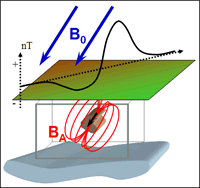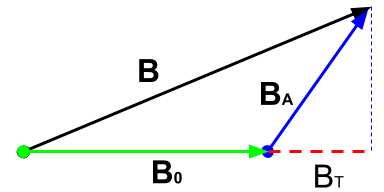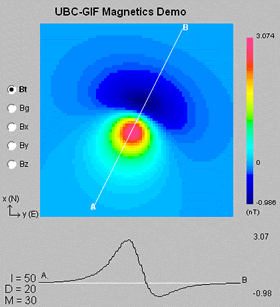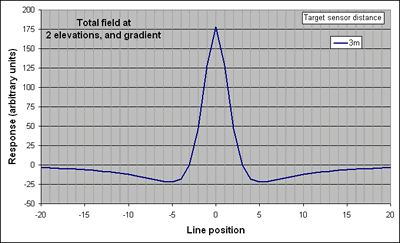# Data¶In this section we present a summary about the different types of magnetic data. Different assumptions are made depending on the type of instrument used during acquisition.

## Magnetic field data¶

As demonstrated in Fig. 40, the magnetic field measured above the surface is a vector quantity. The magnetic field data measured at any location contains the signal from both the source ($$\mathbf{B}_0$$), as well as the response ($$\mathbf{B}_A$$) from magnetized material:

$\mathbf{B} = \mathbf{B}_0 + \mathbf{B}_A\;.$

In ideal cases, magnetic surveys would measure all three components of the field (fluxgate magnetometer). The magnetic field anomaly, the quantity of interest, is readily available by simple subtraction of the inducing field such that:

$\mathbf{B}_A = \mathbf{B} - \mathbf{B}_0 \;.$

The acquisition of three-components data remains challenging however. The orientation of each components needs to corrected in real-time in order to compensate for sensors rotation. Most surveys measure instead the total strength of the field, or Total Magnetic Intensity data:

$|\mathbf{B}| = |\mathbf{B}_0 + \mathbf{B}_A| \;.$

Since we do not know the direction of $$\mathbf{B}_A$$ we assume that the anomalous field is mostly induced and that it’s direction aligns with the Earth’s inducing field $$\mathbf{B}_0$$. This allows us to approximate the Total Magnetic Anomaly datum:

$B_T = \mathbf{B}_A \cdot \mathbf{\hat B}_0 \;,$This assumption holds as long as $$\mathbf{\hat B}_0 \gg \mathbf{\hat B}_A$$, which is valid in most cases considering the strength of the Earth’s field.Total field strength, 10m from target. Total field strength, 11m from target. Vertical gradient, no change in scale. Vertical gradient, full scale.Total field line profile at 3m elevation Total field line profile at 4m elevation Difference between these two - the vertical gradient All three on one image. Above: Line profiles over a vertically oriented dipole. Left: The total field and gradient responses above a dipolar magnetic anomaly are shown for a location where inclination is 50°, and declination is 20°.